# Adding, Subtracting, Multiplying and Dividing Without Using Formulas in Microsoft Excel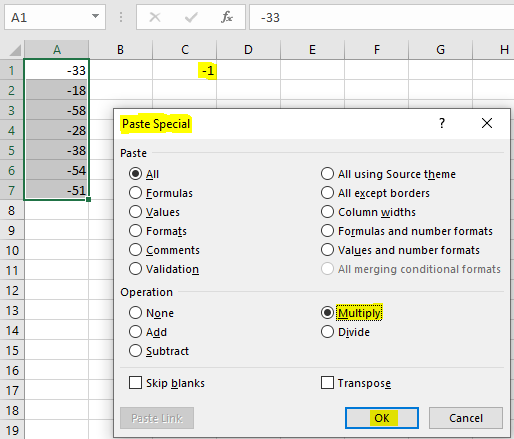If we need to add, subtract, multiply and divide the numbers without using formulas, we can use the Paste Special option in Microsoft Excel.

Paste Special: You can use the Paste Special feature to paste specific cell content or attributes such as formula, formatting, comments, etc.

Let’s take an example to understand that how we can add, subtract, multiply and divide the numbers without using formulas in Excel

Cell A1 contains number 25 and cell B1 contains the number 60. We need to add 25 and 60 without using any formulae.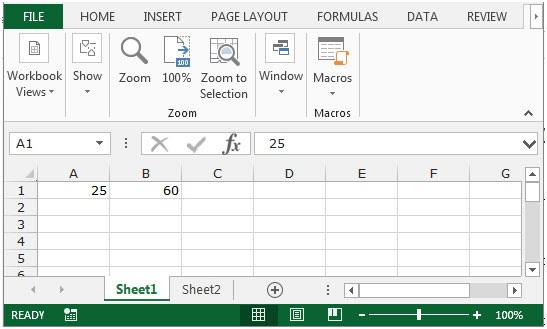• Select the cell A1.
• Copy the cell by pressing the key Ctrl+C on your keyboard.
• Select the cell B1, right click with the mouse.
• From the shortcut menu, select the Paste Special option.
•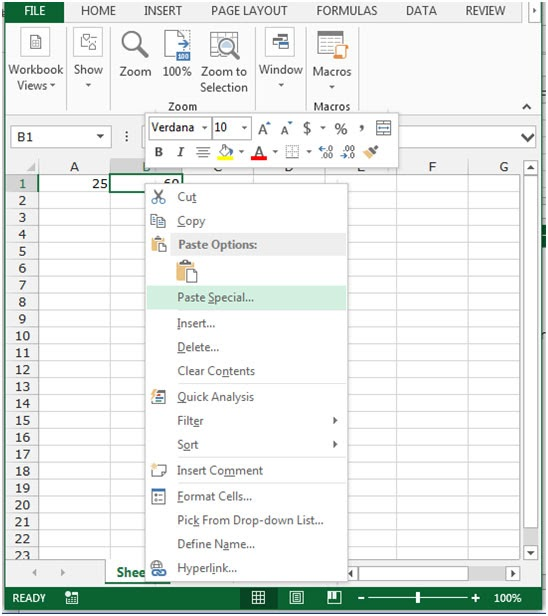• The Paste Special dialog box will appear.
•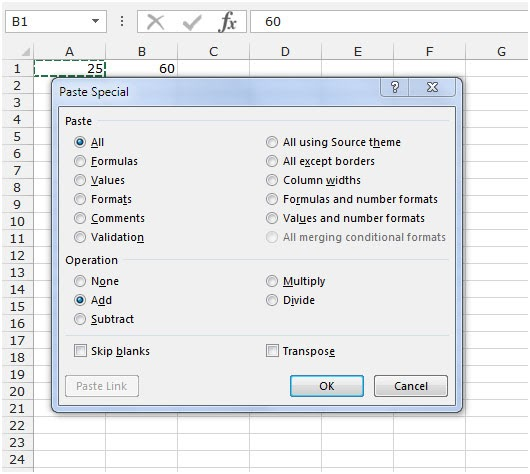• Click on Add in the Operation section.
• Click on OK.
• You will find the result as the addition of both numbers in cell B1.  Hence we get 85 in cell B1 which is 25+60.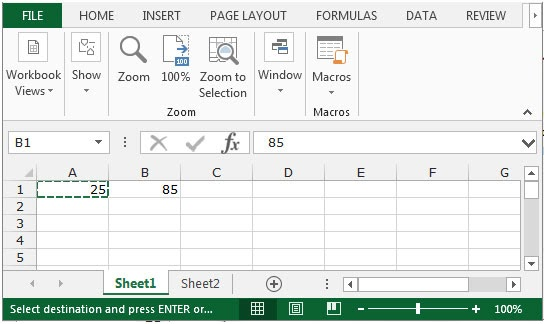Subtract without using formula

• Select the cell A1.
• Copy the cell by pressing the key Ctrl+C on your keyboard.
• Select the cell B1, right click with the mouse.
• From the shortcut menu, select the Paste Special option.
• The Paste Special dialog box will appear.
• Click on Subtract in the Operation section.
•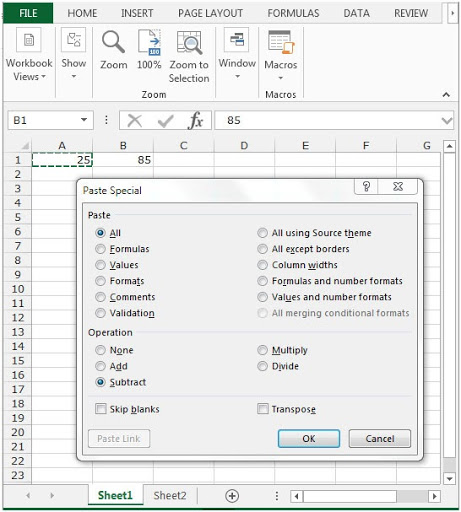• Click on OK.
• The result in cell B1 will be the value of B1-A1 which is 85-25 = 60.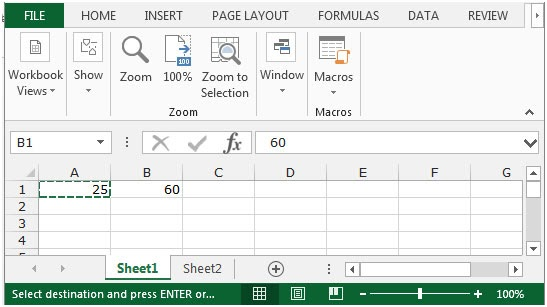Multiply without using formula

• Select the cell A1.
• Copy the cell by pressing the key Ctrl+C on your keyboard.
• Select the cell B1, right click with the mouse.
• From the shortcut menu, select the Paste Special option.
• The Paste Special dialog box will appear.
• Click on Multiply in the Operation section.
•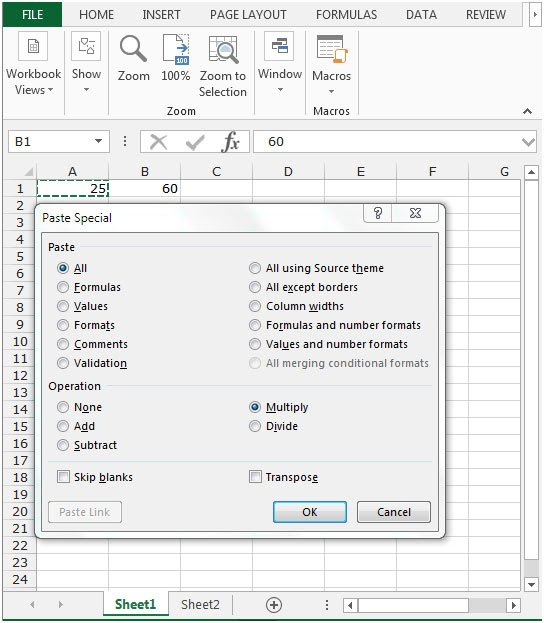• Click on OK.
• The result in cell B1 will be the multiplication of A1 and B1 which is 1500.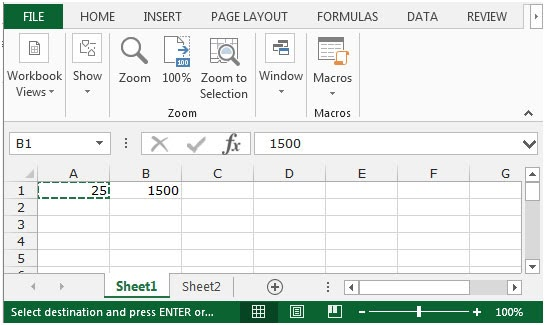Divide without using formula

• Select the cell A1.
• Copy the cell by pressing the key Ctrl+C on your keyboard.
• Select the cell B1, right click with the mouse.
• From the shortcut menu select the Paste Special option.
• The Paste Special dialog box will appear.
• Click on Divide in the Operation section.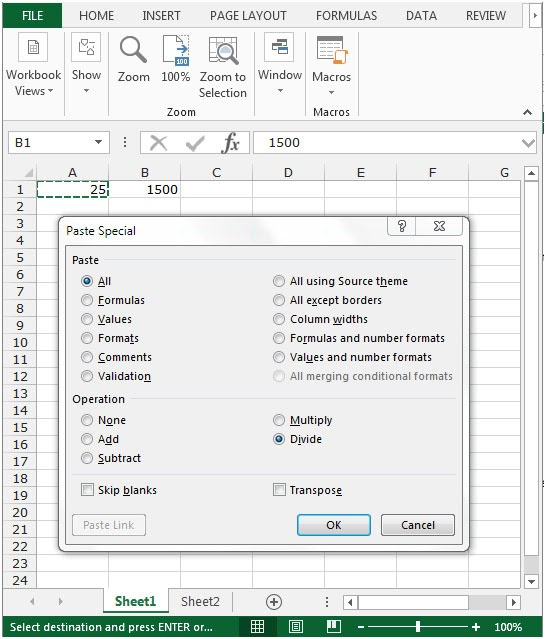• Click on OK.
• The result in cell B1 will be cell B1 divided by cell A1 which is 1500/25 = 60.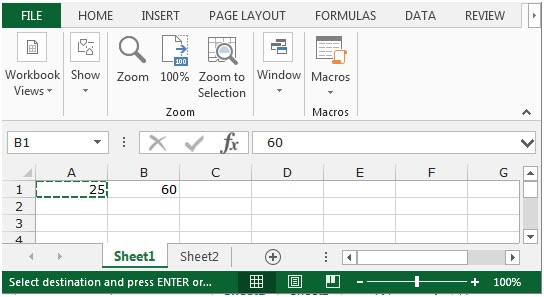This is the way you can Add, Subtract, Multiply and Divide the numbers without using Formulas.

How to perform basic functions on range ?

Usually we have to take one another column and use the formula =value * multiply_value and copy and paste this formula to other cells using the Ctrl + D. Paste option also allows you to perform operation over when pasting values.

Calculation operation paste shortcut

Paste Special allows you to perform basic operations like addition, subtraction, multiplication and division. This are options under Operation

1. None
3. Substract
4. Multiply
5. Divide

You just need to follow steps as stated

• In any cell, type -1.
• Select the cell and press Ctrl+C to copy.
• Select the range to be multiplied by -1.
• Press Shift+F10 or right-click, and then select Paste
• Special from the shortcut menu.
• Select the Multiply option button, and then click OK.

Example :

All of these might be confusing to understand. Let's understand how to use the Paste Special using an example. Here we have a range of numbers and need to multiply that range with -1.

• In B2 cell, type -1.
• Select the cell and press Ctrl+C to copy.
• Select the A1:A7 range.
• Press Shift+F10 or right-click, and then select Paste
• Special from the shortcut menu.
• Select the Multiply option button, and then click OK.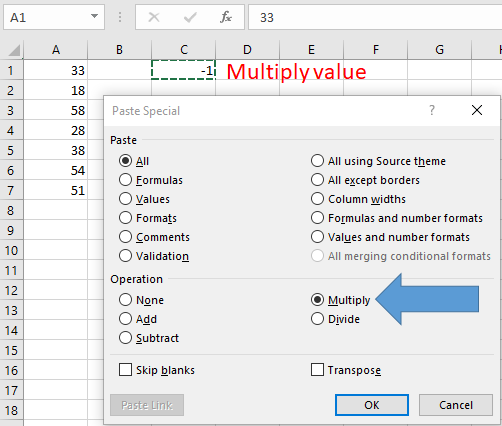As you can see the paste special dialog box. Click OK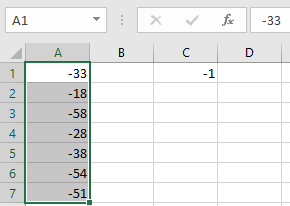Here are all the observational notes using How to Multiply a Range by -1 in One Operation in Excel.

Notes :

1. This option doesn't allow you to change the multiply value with changing the number in the cell. Then you need to use cell reference.
2. Cannot perform other operations other than addition, multiplication, subtraction and division.

Hope this article about How to Multiply a Range by -1 in One Operation in Excel is explanatory. Find more articles on pasting values or formulas and related Excel operation here. If you liked our blogs, share it with your friends on Facebook. And also you can follow us on Twitter and Facebook. We would love to hear from you, do let us know how we can improve, complement or innovate our work and make it better for you. Write to us at info@exceltip.com.

Related Articles :

SUM if date is between : Returns the SUM of values between given dates or period in excel.

Sum if date is greater than given date : Returns the SUM of values after the given date or period in excel.

2 Ways to Sum by Month in Excel : Returns the SUM of values within a given specific month in excel.

How to Sum Multiple Columns with Condition : Returns the SUM of values across multiple columns having condition in excel.

50 Excel Shortcut’s to Increase Your Productivity : The Excel shortcuts enhance the efficiency of work a lot. These 50 shortcuts can increase your productivity exceptionally on Excel

Excel Insert Row Shortcut : These shortcuts will help you insert single and multiple rows quickly.

Excel Shortcut Keys for Merge and Center : This Merge and Center shortcut helps you quickly merge and unmerge cells.

How to use wildcards in excel : Count cells matching phrases using the wildcards in excel.

Popular Articles :

How to use the IF Function in Excel : The IF statement in Excel checks the condition and returns a specific value if the condition is TRUE or returns another specific value if FALSE.

How to use the VLOOKUP Function in Excel : This is one of the most used and popular functions of excel that is used to lookup value from different ranges and sheets.

How to Use SUMIF Function in Excel : This is another dashboard essential function. This helps you sum up values on specific conditions.

How to use the COUNTIF Function in Excel : Count values with conditions using this amazing function. You don't need to filter your data to count specific values. Countif function is essential to prepare your dashboard.

1.